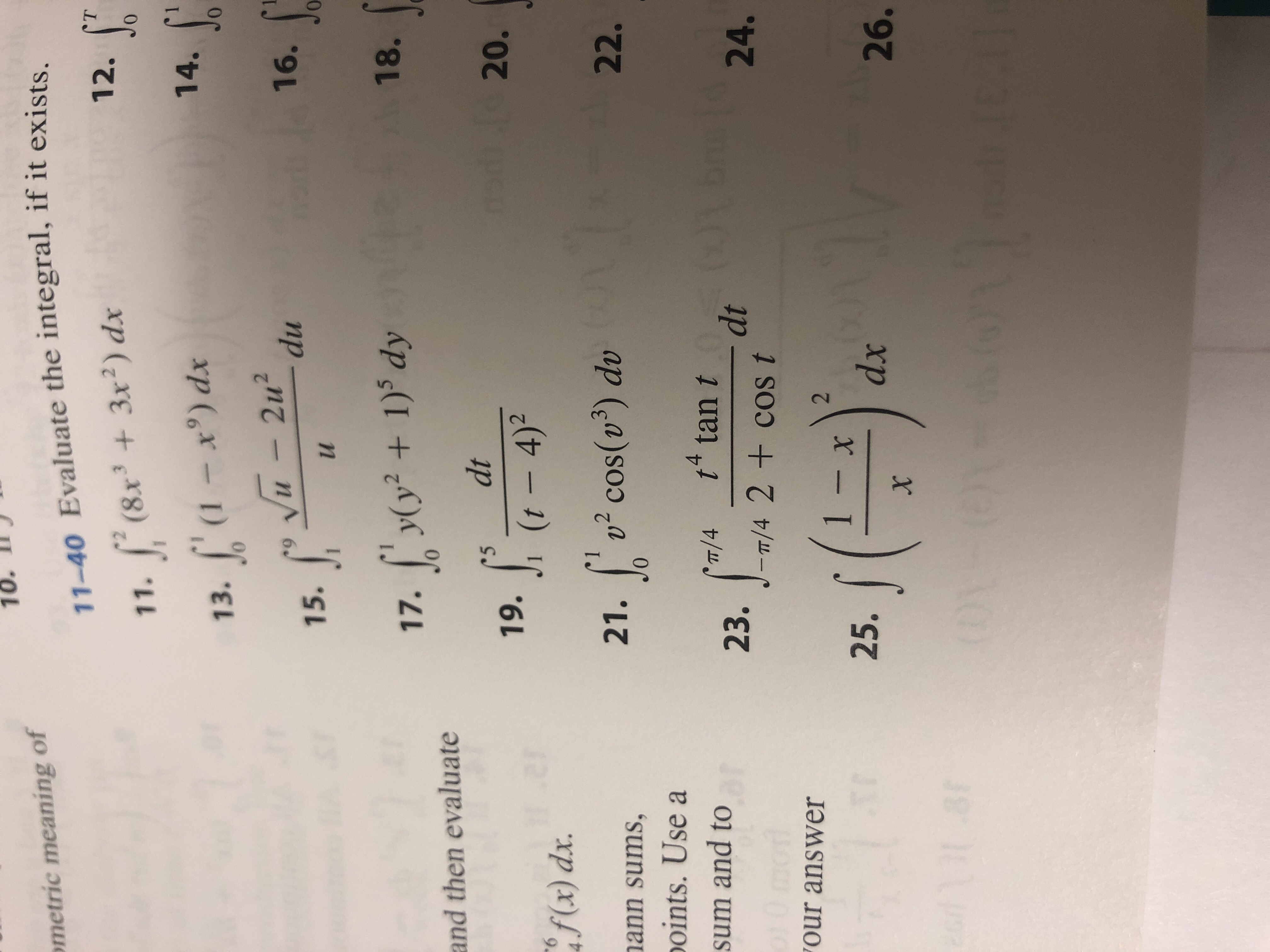# 10.ometric meaning of11-40 Evaluate the integral, if it existsT12.(8.x33x2) dx011.14.(1 - x°) dx013.Vu- 2udu16.015.A18.yy2 + 1) dy17.and then evaluatedtSC520.19.(t 4)2.27f(x) dx.2 Cos() dv22.21.nann sums,oints. Use at tan tdt- 1/4 2 + cos t'/4sum and to23.24.TTOur answer2- Xdx25.26.X

Question
1 views

Number 21help_outlineImage Transcriptionclose10. ometric meaning of 11-40 Evaluate the integral, if it exists T 12. (8.x33x2) dx 0 11. 14. (1 - x°) dx 0 13. Vu- 2u du 16. 0 15. A18. yy2 + 1) dy 17. and then evaluate dt SC 5 20. 19. (t 4)2 .27 f(x) dx. 2 Cos() dv 22. 21. nann sums, oints. Use a t tan t dt - 1/4 2 + cos t '/4 sum and to 23. 24. TT Our answer 2 - X dx 25. 26. X fullscreen
check_circle

Step 1

Calculation:

Compute the given integral using Substitution method.

Let u = v3, then du = 3v2dv.

Obtain the change in limit as follo...

### Want to see the full answer?

See Solution

#### Want to see this answer and more?

Solutions are written by subject experts who are available 24/7. Questions are typically answered within 1 hour.*

See Solution
*Response times may vary by subject and question.
Tagged in
MathCalculus

### Other